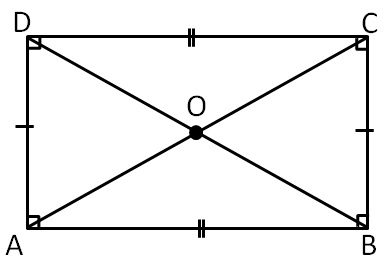Rhombus, Rectangle, Square

Chapter 3 Class 8 Understanding Quadrilaterals
Concept wiseRectangle is a parallelogram where

all angles are 90°

Properties of Rectangle:

Since it is a parallelogram,

it will have all its properties

In parallelogram

• Opposite sides are equal
• Opposite angles are equal
• Diagonals bisect each other
• Sum of adjacent angles is 180°

Other properties are

Diagonals are of equal length

i.e. AC = BD

Learn in your speed, with individual attention - Teachoo Maths 1-on-1 Class